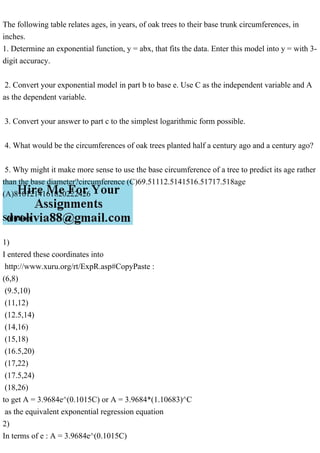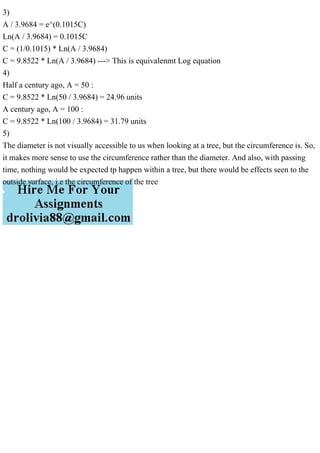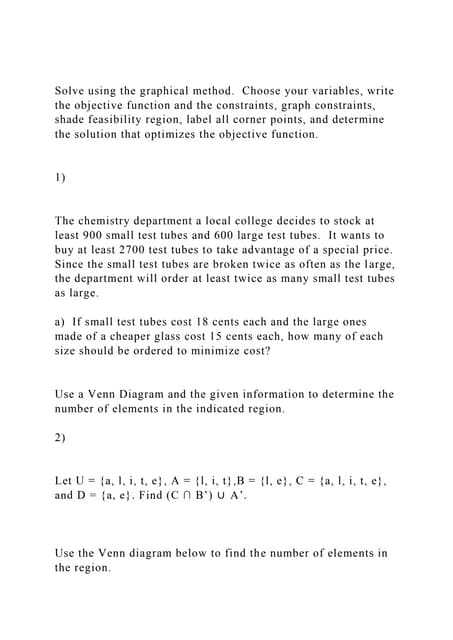Publicité

# The following table relates ages, in years, of oak trees to their ba.pdf

2 Apr 2023Prochain SlideShareSolve using the graphical method. Choose your variables, write th.docx
Chargement dans ... 3
1 sur 2
Publicité

### The following table relates ages, in years, of oak trees to their ba.pdf

1. The following table relates ages, in years, of oak trees to their base trunk circumferences, in inches. 1. Determine an exponential function, y = abx, that fits the data. Enter this model into y = with 3- digit accuracy. 2. Convert your exponential model in part b to base e. Use C as the independent variable and A as the dependent variable. 3. Convert your answer to part c to the simplest logarithmic form possible. 4. What would be the circumferences of oak trees planted half a century ago and a century ago? 5. Why might it make more sense to use the base circumference of a tree to predict its age rather than the base diameter?circumference (C)69.51112.5141516.51717.518age (A)8101214161820222426 Solution 1) I entered these coordinates into http://www.xuru.org/rt/ExpR.asp#CopyPaste : (6,8) (9.5,10) (11,12) (12.5,14) (14,16) (15,18) (16.5,20) (17,22) (17.5,24) (18,26) to get A = 3.9684e^(0.1015C) or A = 3.9684*(1.10683)^C as the equivalent exponential regression equation 2) In terms of e : A = 3.9684e^(0.1015C)
2. 3) A / 3.9684 = e^(0.1015C) Ln(A / 3.9684) = 0.1015C C = (1/0.1015) * Ln(A / 3.9684) C = 9.8522 * Ln(A / 3.9684) ---> This is equivalenmt Log equation 4) Half a century ago, A = 50 : C = 9.8522 * Ln(50 / 3.9684) = 24.96 units A century ago, A = 100 : C = 9.8522 * Ln(100 / 3.9684) = 31.79 units 5) The diameter is not visually accessible to us when looking at a tree, but the circumference is. So, it makes more sense to use the circumference rather than the diameter. And also, with passing time, nothing would be expected tp happen within a tree, but there would be effects seen to the outside surface, i.e the circumference of the tree
Publicité